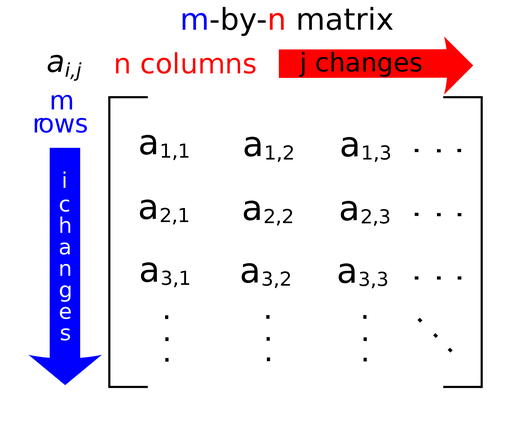# Glossary

## Matrix

A matrix is simply a rectangular table of values; we say that a matrix with $m$ rows and $n$ columns has dimensions $m \times n$. Given a matrix $A$, we write $A_{i, j}$ (or sometimes $a_{i, j}$ to indicate the value found at the intersection of row $i$ and column $j$, as shown in the figure below.

It may be helpful to think of the rows of an $m \times n$ matrix $A$ as a collection of $m$ arrays, each having length $n$.